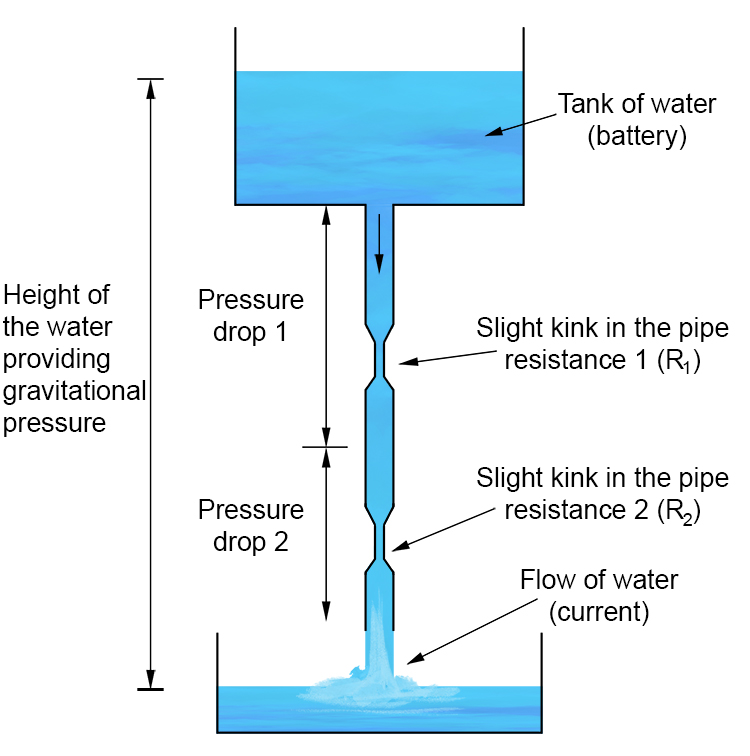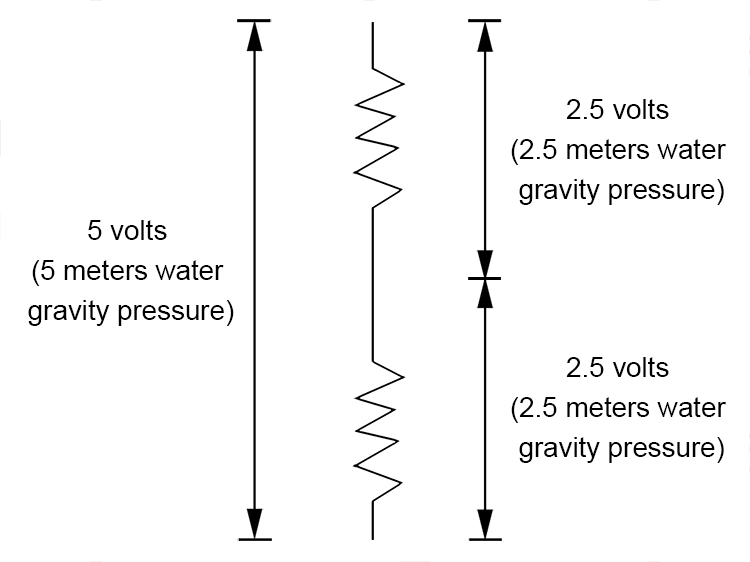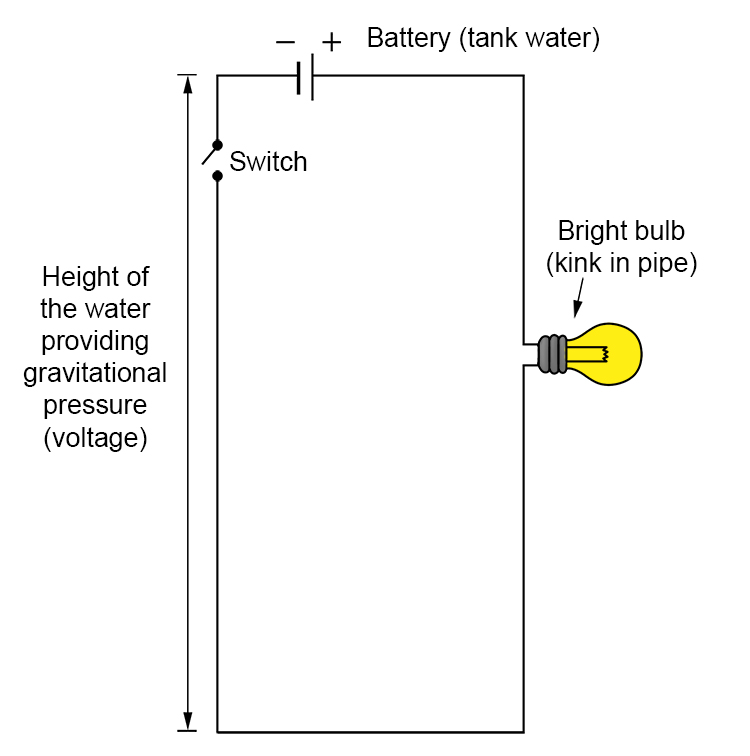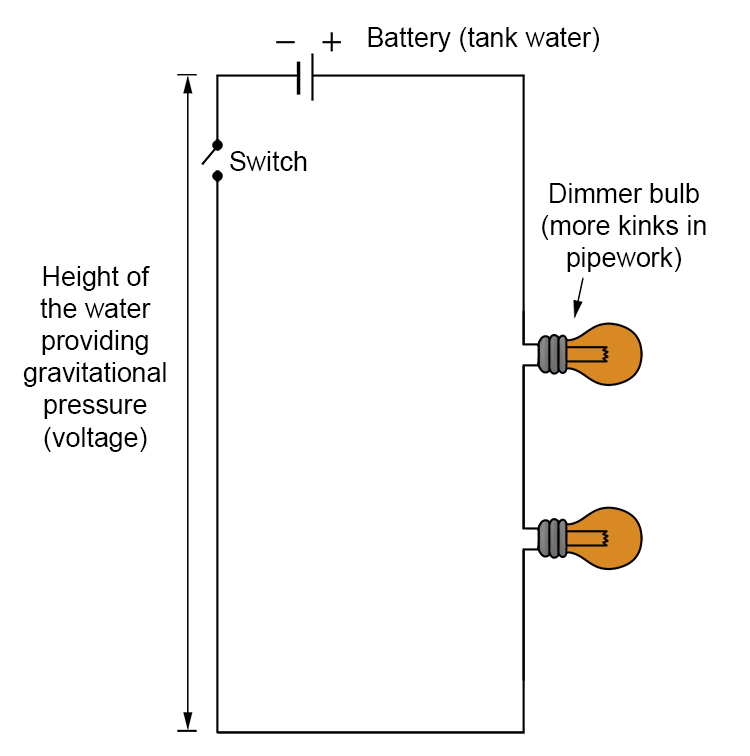# Series Resistance Water Analogy

To the picture on the previous page we should now add another kink to the pipe (resistance).This example diagram provides a visual explanation of the rules you need to remember series resistance.

1/ The overall current reduces, but the current is the same in all parts of the circuit. Current is not "used up" along the circuit, so remains constant.

You can calculate the total voltage of the current by dividing the total voltage of the battery by the total resistance.

 i.e. V = IR therefore I = \frac{V}{R_{Total}} and R_{Total} = R_1 + R_2

2/ Total voltage remains the same, but it's shared amongst components.

The total voltage from the battery (tank) is shared between the components (resistances).

If the battery provides 5 volts (5 meters of water gravity) and there are two lamps (two resistances or kinks in the pipe) each lamp will get 2.5 volts.3/ Adding resistance reduces the current and all the lights become dimmer.

If you add a lamp (resistance) to a series circuit, the total resistance increases and hence current decreases.I (current or water flow) must decrease even though all the components get the same current where ever you measure it.

Example

I=\frac{V}{R_T_o_t_a_l}=\frac{5volts}{1ohm}=5amps

and now add a light bulb (more kinks/resistance) in series.

I=\frac{V}{R_T_o_t_a_l}=\frac{5volts}{1ohm+1ohm}=\frac{5}{2}=2.5amps

So the light bulb must get dimmer or in the water analogy, the water flow reduces speed through the pipe.Adding another bulb in the series.Adding another bulb in the series will make both bulbs dimmer.